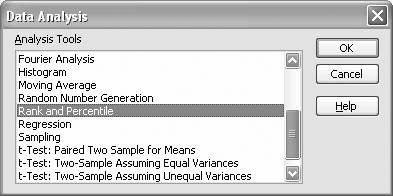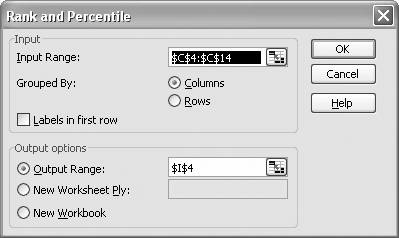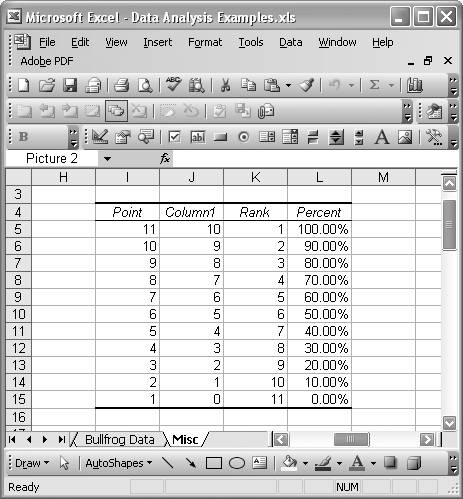# Ranking and Percentiles

Problem

You need to compute certain percentiles of a set of data and you'd also like to compute the rank of certain values in the dataset.

Solution

Use the built-in functions PERCENTILE, RANK, and PERCENTRANK. Or you can use the Rank and Percentile tool available in the Analysis ToolPak.

Discussion

By way of example, let's assume your dataset consists of the values 1, 3, 5, 7, 9, 2, 4, 6, 8, 10, and 0. Let's also assume this dataset resides on a spreadsheet in the cell range C18:C28. (This is a rather simple dataset for example purposes; in practice your dataset can be anything and it need not be sorted.)

To compute the 25% percentile, use the formula =PERCENTILE(C18:C28,0.25), which returns a value of 2.5. To compute the 95% percentile, use =PERCENTILE(C18:C28,0.95), which returns a value of 9.5 as expected.

To compute the rank of a given value, use the formula =RANK(2,C18:C28,1). The rank of the value 2 in this dataset is 3, assuming the dataset is sorted in ascending order. If you want to compute the rank of a value as though the dataset were in descending order, then use a value of 0 for the third argument in the call to RANK.

If you'd like to compute the rank of a value in percentage terms, then use the PERCENTRANK function. For example, =PERCENTRANK(C18:C28,2,2) returns the rank of the value 2 as 20%. The second argument in this function call represents the value whose rank is sought. The third argument is the number of significant digits for the result (in this case, two significant digits).

Instead of using these built-in functions, you could use the Analysis ToolPak's Rank and Percentile tool. Select Tools [Image_Link]/images/U2192.jpg border=0> Data Analysis from the main menu bar to open the Data Analysis dialog box shown in Figure 5-13.

Select "Rank and Percentile" from the list and press OK to open the "Rank and Percentile" dialog box shown in Figure 5-14.

In the Input Range field, type (or select from your spreadsheet) the cell range containing the input dataset you'd like to rank. You can also specify the output location, as shown in Figure 5-14.

Figure 5-13. Data Analysis dialog boxFigure 5-14. Rank and Percentile tool dialog boxFor the simple example dataset discussed earlier, the Rank and Percentile tool returns the results shown in Figure 5-15.

The ranks are computed assuming the data is sorted in descending order. The first column in the resulting table contains an index for each data point. The second column contains the raw value of the data point. The third column contains the corresponding rank. The last column contains the percentage rank.

In the event of ties (that is, where two or more values in the dataset are the same), you may want to use the correction recommended in Excel's help file for computing ranks. The formula to use is =[COUNT(data)+1-RANK(value, data, 0)-RANK(value, data, 1)]/2. In this formula, data is a cell reference containing the input dataset and value is the value for which you want to find a rank. See the Excel help topic for the RANK function for more information. To the best of my knowledge, the Rank and Percentile tool in the Analysis ToolPak does not handle ties. So if your data contains ties and you need to correct for this, you should use the RANK function instead.

Figure 5-15. Rank and Percentile resultsExcel Scientific and Engineering Cookbook (Cookbooks (OReilly))
ISBN: 0596008791
EAN: 2147483647
Year: N/A
Pages: 206
Authors: David M Bourg